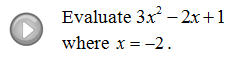## Algebra

Showing posts with label evaluate. Show all posts
Showing posts with label evaluate. Show all posts

### Introduction to Functions

You may have noticed that we have been evaluating or "plugging-in" lots of numbers to find the corresponding y-values.  We have been doing this to find points on the graph (x, y).  This process can be streamlined using function notation.This new notation above is read, "y is equal to f of x".  A function is a rule that uniquely assigns one output to every one input. So, at this point we can think of our non-vertical lines as functions.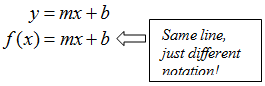The domain of a function is the set of inputs, usually the x-values.  The range of a function is the set of outputs, usually the y-values.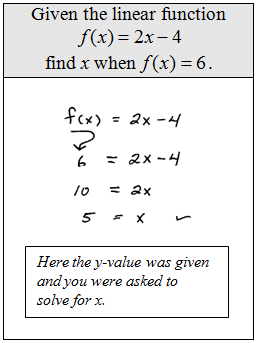Evaluate the given function.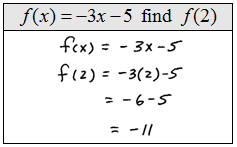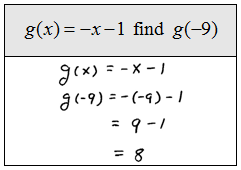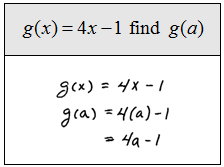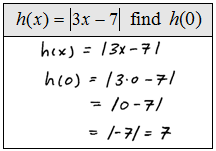Find x.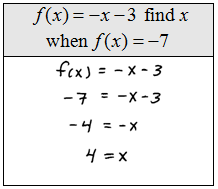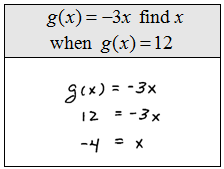Do not let the function notation discourage you, it takes some time to get used to.  The main thing to remember is that y = f(x); sometimes x is given and sometimes y or f(x) is given.

Graph the given linear function.Word Problem. Bill has a popular software company which sells copies of its program for \$149.  If the initial start up cost for the company was \$10,000 and it costs \$12 to produce each copy:
a.  Find a cost function C(x) that models this business.
b.  Find a revenue function R(x) that models this business.
c.  Find the profit function P(x) using your functions above.
d.  Find the profit when 1000 programs are produced and sold.
e.  Find the number of programs that must be sold to break even.

Solution: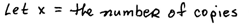a. Cost Function - Include all fixed and variable costs of production.b. Revenue Function - Include all proceeds from sales.c. Profit Function - Revenue less cost of production.d. Here x = 1,000 programs produced and sold.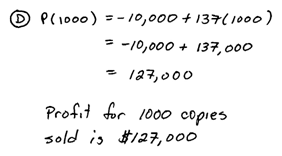e. Break Even - Occurs when profit is equal to zero.Video Examples on YouTubeIntro to Functions PlayList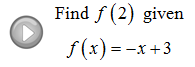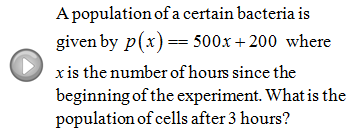### Introduction to Polynomials

Before beginning this section we first make a few definitions. Some of the terms have already been defined but it does not hurt to go over them again. First recall that algebraic expressions such as,
are called terms. The term,
has a coefficient −2 and a variable part a^2 b. A polynomial is any sum or difference of algebraic terms. A polynomial with only one term is called a monomial. A polynomial with 2 terms is called a binomial and one with 3 terms is called a trinomial.  We will not use any other special names for polynomials with more terms.
It is common practice to express polynomials in descending order from largest exponent down to the constant term. The constant term, or the term with no variable part, can be thought of as the coefficient of the x^0 term.
The degree of a polynomial with one variable is the largest exponent.
Evaluating expressions involves replacing the variable with the appropriate numerical value. In other words, plug in the values and use the order of operations to calculate the answer.
Evaluate.
Simplifying expressions first saves steps when evaluating and the results will be the same.
We can use function notation to evaluate. Do not let the notation get in the way of your ability to do these types of problems. The idea is the same, just substitute in the appropriate values.

Evaluate.
We will often run into problems where the graph is given instead of the algebraic equation. In this case, read the graph and answer the question.

Given the graph of the function.
Given the graph of the function.
Projectile Problem: A projectile is fired from the ground with an initial velocity of 64 feet per second. The height of the projectile in feet after t seconds is given by the function
with the following graph:
a. Use the graph to determine how much time it takes to reach the maximum height.
b. How much time will it take to hit the ground?
c. What times will the projectile be at 60 feet?
d. Use the function  to determine the height of the projectile at t = 1 second.

Geometry Problem: The function
gives the volume of a sphere given its radius r. Use the function to calculate the volume of a sphere of radius 6 centimeters. (Use 3.14 as an approximation for pi.)
Projectile Problem: A projectile is fired upwards from the roof of a 256 foot building with an initial velocity of 96 feet per second. The height of the projectile in feet after t seconds is given by
whose graph is given:
a. How much time does it take to reach a maximum height?
b. What is the maximum height?
c. How much time does it take for the projectile to hit the ground?
d. Use the function to determine the height after 6 seconds.